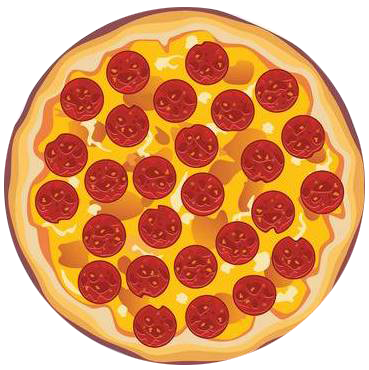•• Merch
• Donate
• SAT Prep

IntroductionIntroduction# Multiplying Fractions

To help us better understand what happens when we multiply fractions, we like to imagine them as pizzas or legos.

Pick whichever you vibe with more:

INTRO
When we’re given a pair of fractions to multiply, like , the second fraction tells us how many parts we need to split the first fraction into, and how many of those parts we get to keep.
means that we need to take , split it into equal parts, and keep of those parts:Visualizing the multiplication helps us understand why a fraction’s size changes when it’s multiplied by another number. But, if we had to draw this out every time we multiply a fraction, we’d probably lose our minds 🤯.
Luckily, there’s a quick and simple process we can use to multiply any set of fractions! 🙌🏿🙌🏻🙌🏼
All we need to do is multiply across the top and multiply across the bottom.
Check out our
Calculator
or explore our
Lesson
and
Practice

CALCULATOR

KEY STEPS

## How to Multiply Fractions

### Step 1. Make sure both numbers are fractions.

If multiplying a fraction by a whole number, convert the whole number to a fraction by placing it over . Otherwise, move onto Step 2.

### Step 4. Simplify (if needed).

LESSON
Multiplying Fractions

## Multiplying Fractions

To multiply fractions, all we need to do is multiply across top and multiply across the bottom.To understand what it means to multiply two fractions, we can start by imagining the first fraction as a pizza or lego.
The bottom of the second fraction tells us how many parts we need to split the first fraction into, and the top of the second fraction tells us how many of those parts we get to keep.
So if we multiply a fraction by another fraction that is the fraction's size increases.Now that we know what happens when we multiply fractions, let’s check out some examples of how to multiply fractions using our simple steps!
PRACTICE
Multiplying Fractions

## Practice: Multiplying Fractions

Question 1 of 10:

### Step 1. Make sure both numbers are fractions.

Since both numbers are already fractions, we can just move on to Step 2.

CONCLUSION
Incredible job, look at you go! Thanks for checking out this lesson ☺️🙏. Where to next?Leave Feedback